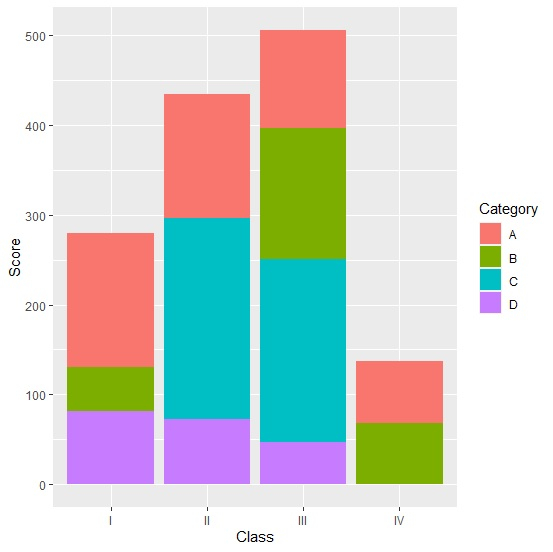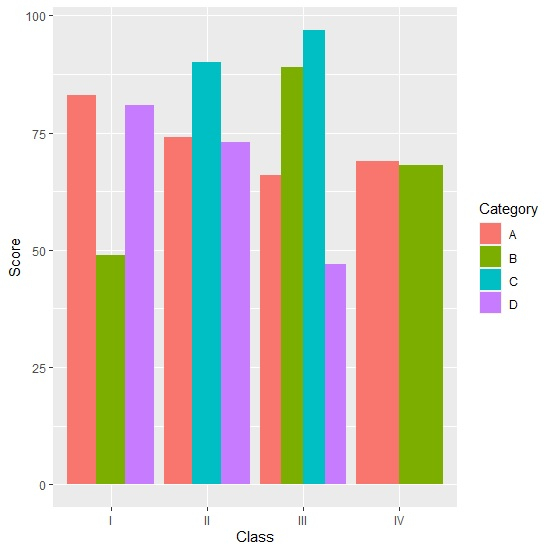# How to create a stacked bar plot with vertical bars in R using ggplot2?

Traditionally, the stacked bar plot has multiple bars for each level of categories lying upon each other. But this visual can be changed by creating vertical bars for each level of categories, this will help us to read the stacked bar easily as compared to traditional stacked bar plot because people have a habit to read vertical bars.

Consider the below data frame −

## Example

Live Demo

set.seed(999)
Class<-sample(c("I","II","III","IV"),20,replace=TRUE)
Category<-sample(LETTERS[1:4],20,replace=TRUE)
Score<-sample(41:100,20)
df<-data.frame(Class,Category,Score)
df

## output

   Class Category Score
1  II     D        47
2  III    C        88
3  I      C        83
4  IV     B        67
5  IV     D        61
6  I      D        56
7  III    C        74
8  I      C        54
9  II     D       100
10 III    B        43
11 II     A        77
12 III    A        72
13 I      C        92
14 IV     C        81
15 I      C        49
16 IV     D        97
17 I      D        91
18 IV     D        73
19 I     A         59
20 I     B         75

Loading ggplot2 and creating a stacked bar chart with bars on top of each other −

## Example

library(ggplot2) ggplot(df,aes(Class,Score,fill=Category))+geom_bar(stat="identity")

## outputCreating a stacked bar chart with vertical bars −

## Example

ggplot(df,aes(Class,Score,fill=Category))+geom_bar(stat="identity",position="dodge")

## output